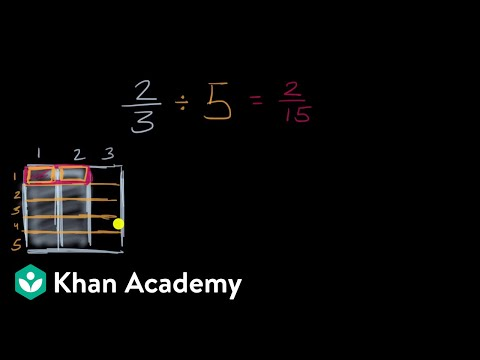Video

# Dividing mixed numbers (Full video)

Description: Sal divides 4 4/5 by 1 1/2. Your going to get, you add the numerators, you get 24 fifths, 24 fifths, another way to think about it is take this denominator, take the fifths, multiply by four, you get 20 fifths, plus the four fifths that you already have is 24 fifths and so this is the same things as 24 fifths divided by, use the same idea, one and a half is the same thing as one plus one half, one is the same thing as two halves plus one half and so that's going to be, add the numerators, that's going to be three halves, so just like this, we're able to rewrite our expression as 24 fifths divided by three halves and now the key realization is is that that is the same thing as 24 fifths times the reciprocal of three halves, so times, pause the video, what's the reciprocal of three halves?

### Other videos you might be interested in### Dividing Fractions Word Problems (Full video)

#### Student Achievement Partners